# Monads and Monoids by Oleksiy Dyagilev

Oct. 7, 2015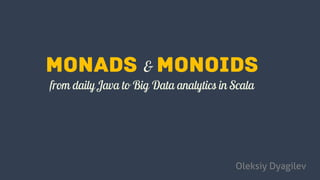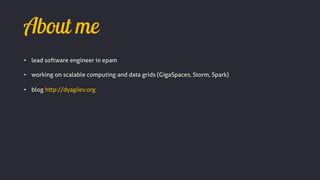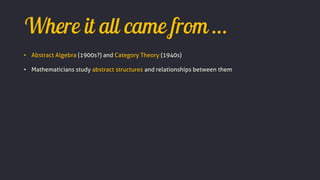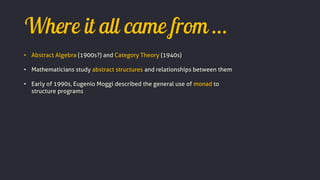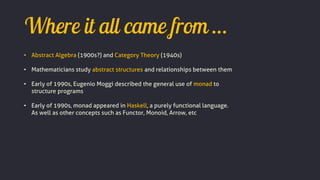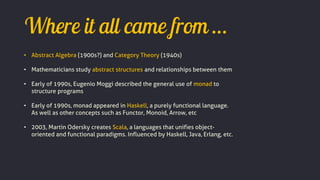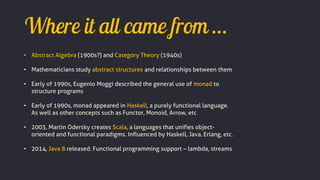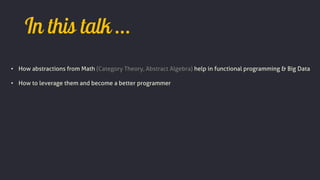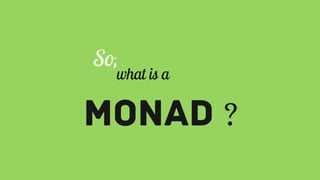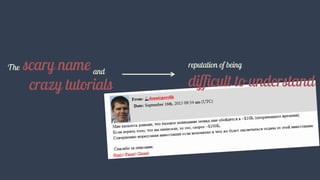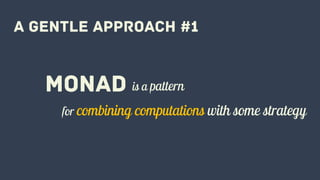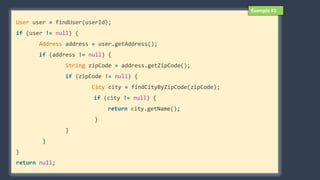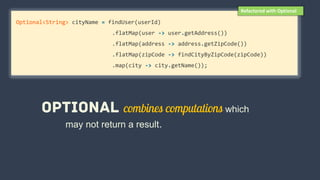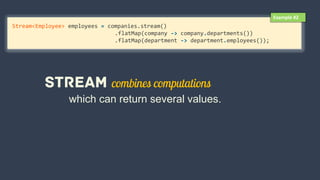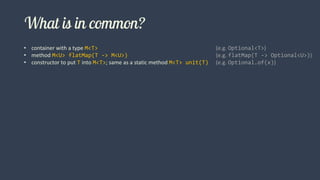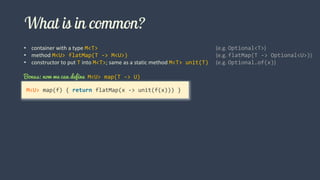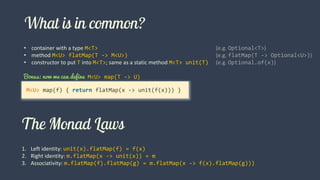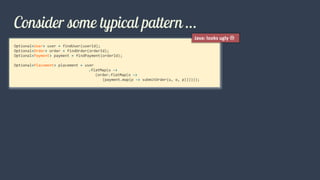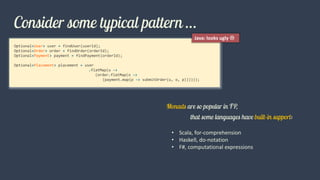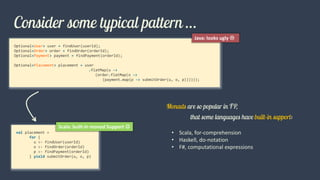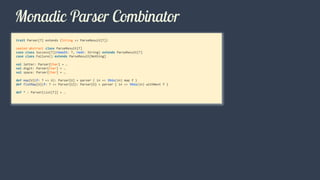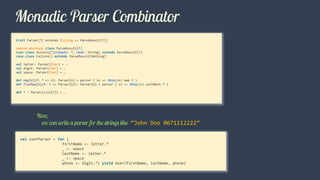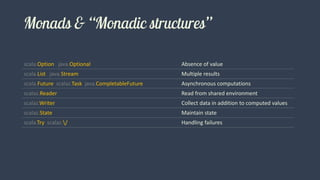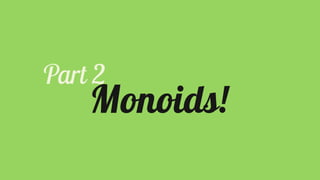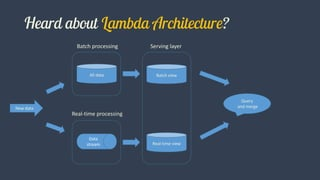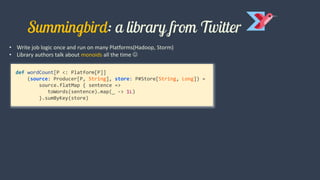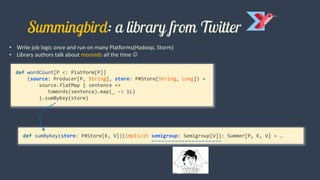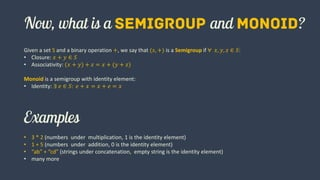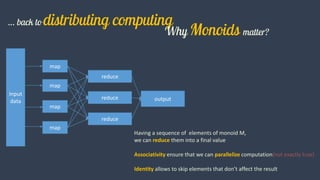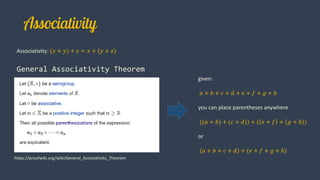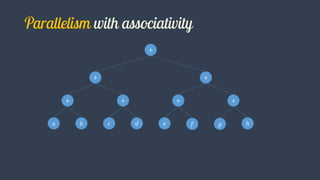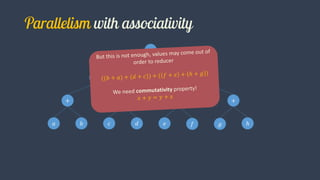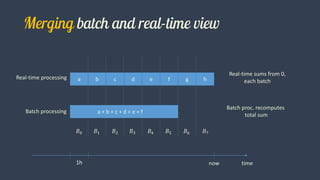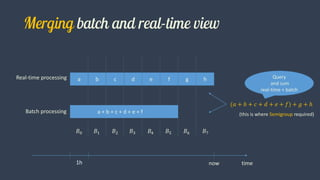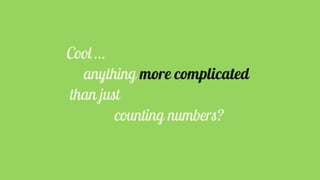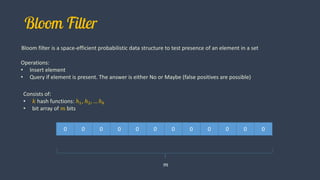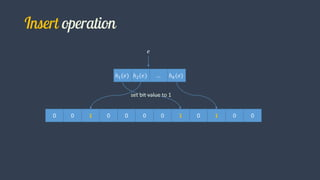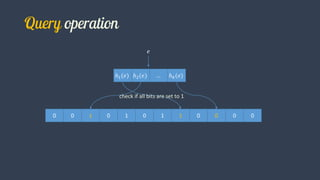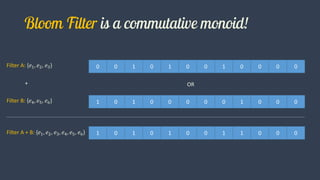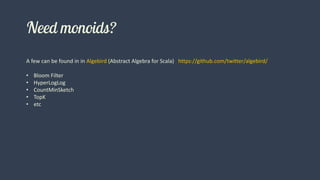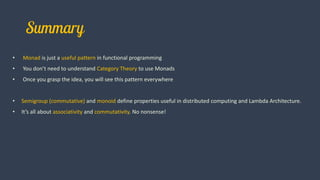1 of 50

### Monads and Monoids by Oleksiy Dyagilev

• 1. Oleksiy Dyagilev
• 2. • lead software engineer in epam • working on scalable computing and data grids (GigaSpaces, Storm, Spark) • blog http://dyagilev.org
• 3. • Abstract Algebra (1900s?) and Category Theory (1940s) • Mathematicians study abstract structures and relationships between them
• 4. • Abstract Algebra (1900s?) and Category Theory (1940s) • Mathematicians study abstract structures and relationships between them • Early of 1990s, Eugenio Moggi described the general use of monad to structure programs
• 5. • Abstract Algebra (1900s?) and Category Theory (1940s) • Mathematicians study abstract structures and relationships between them • Early of 1990s, Eugenio Moggi described the general use of monad to structure programs • Early of 1990s, monad appeared in Haskell, a purely functional language. As well as other concepts such as Functor, Monoid, Arrow, etc
• 6. • Abstract Algebra (1900s?) and Category Theory (1940s) • Mathematicians study abstract structures and relationships between them • Early of 1990s, Eugenio Moggi described the general use of monad to structure programs • Early of 1990s, monad appeared in Haskell, a purely functional language. As well as other concepts such as Functor, Monoid, Arrow, etc • 2003, Martin Odersky creates Scala, a languages that unifies object- oriented and functional paradigms. Influenced by Haskell, Java, Erlang, etc.
• 7. • Abstract Algebra (1900s?) and Category Theory (1940s) • Mathematicians study abstract structures and relationships between them • Early of 1990s, Eugenio Moggi described the general use of monad to structure programs • Early of 1990s, monad appeared in Haskell, a purely functional language. As well as other concepts such as Functor, Monoid, Arrow, etc • 2003, Martin Odersky creates Scala, a languages that unifies object- oriented and functional paradigms. Influenced by Haskell, Java, Erlang, etc. • 2014, Java 8 released. Functional programming support – lambda, streams
• 8. • How abstractions from Math (Category Theory, Abstract Algebra) help in functional programming & Big Data • How to leverage them and become a better programmer
• 17. User user = findUser(userId); if (user != null) { Address address = user.getAddress(); if (address != null) { String zipCode = address.getZipCode(); if (zipCode != null) { City city = findCityByZipCode(zipCode); if (city != null) { return city.getName(); } } } } return null; Example #1
• 18. Optional<String> cityName = findUser(userId) .flatMap(user -> user.getAddress()) .flatMap(address -> address.getZipCode()) .flatMap(zipCode -> findCityByZipCode(zipCode)) .map(city -> city.getName()); which may not return a result. Refactored with Optional
• 19. Stream<Employee> employees = companies.stream() .flatMap(company -> company.departments()) .flatMap(department -> department.employees()); Example #2 which can return several values.
• 20. • container with a type M<T> (e.g. Optional<T>) • method M<U> flatMap(T -> M<U>) (e.g. flatMap(T -> Optional<U>)) • constructor to put T into M<T>; same as a static method M<T> unit(T) (e.g. Optional.of(x))
• 21. • container with a type M<T> (e.g. Optional<T>) • method M<U> flatMap(T -> M<U>) (e.g. flatMap(T -> Optional<U>)) • constructor to put T into M<T>; same as a static method M<T> unit(T) (e.g. Optional.of(x)) M<U> map(f) { return flatMap(x -> unit(f(x))) } Bonus: now we can define M<U> map(T -> U)
• 22. • container with a type M<T> (e.g. Optional<T>) • method M<U> flatMap(T -> M<U>) (e.g. flatMap(T -> Optional<U>)) • constructor to put T into M<T>; same as a static method M<T> unit(T) (e.g. Optional.of(x)) 1. Left identity: unit(x).flatMap(f) = f(x) 2. Right identity: m.flatMap(x -> unit(x)) = m 3. Associativity: m.flatMap(f).flatMap(g) = m.flatMap(x -> f(x).flatMap(g))) M<U> map(f) { return flatMap(x -> unit(f(x))) } Bonus: now we can define M<U> map(T -> U)
• 23. Optional<User> user = findUser(userId); Optional<Order> order = findOrder(orderId); Optional<Payment> payment = findPayment(orderId); Optional<Placement> placement = user .flatMap(u -> (order.flatMap(o -> (payment.map(p -> submitOrder(u, o, p)))))); Java: looks ugly 
• 24. Optional<User> user = findUser(userId); Optional<Order> order = findOrder(orderId); Optional<Payment> payment = findPayment(orderId); Optional<Placement> placement = user .flatMap(u -> (order.flatMap(o -> (payment.map(p -> submitOrder(u, o, p)))))); Java: looks ugly  • Scala, for-comprehension • Haskell, do-notation • F#, computational expressions
• 25. Optional<User> user = findUser(userId); Optional<Order> order = findOrder(orderId); Optional<Payment> payment = findPayment(orderId); Optional<Placement> placement = user .flatMap(u -> (order.flatMap(o -> (payment.map(p -> submitOrder(u, o, p)))))); Java: looks ugly  val placement = for { u <- findUser(userId) o <- findOrder(orderId) p <- findPayment(orderId) } yield submitOrder(u, o, p) Scala: built-in monad Support  • Scala, for-comprehension • Haskell, do-notation • F#, computational expressions
• 27. trait Parser[T] extends (String => ParseResult[T]) sealed abstract class ParseResult[T] case class Success[T](result: T, rest: String) extends ParseResult[T] case class Failure() extends ParseResult[Nothing] val letter: Parser[Char] = … val digit: Parser[Char] = … val space: Parser[Char] = … def map[U](f: T => U): Parser[U] = parser { in => this(in) map f } def flatMap[U](f: T => Parser[U]): Parser[U] = parser { in => this(in) withNext f } def * : Parser[List[T]] = …
• 28. trait Parser[T] extends (String => ParseResult[T]) sealed abstract class ParseResult[T] case class Success[T](result: T, rest: String) extends ParseResult[T] case class Failure() extends ParseResult[Nothing] val letter: Parser[Char] = … val digit: Parser[Char] = … val space: Parser[Char] = … def map[U](f: T => U): Parser[U] = parser { in => this(in) map f } def flatMap[U](f: T => Parser[U]): Parser[U] = parser { in => this(in) withNext f } def * : Parser[List[T]] = … val userParser = for { firstName <- letter.* _ <- space lastName <- letter.* _ <- space phone <- digit.*} yield User(firstName, lastName, phone) “John Doe 0671112222”
• 29. scala.Option java.Optional Absence of value scala.List java.Stream Multiple results scala.Future scalaz.Task java.CompletableFuture Asynchronous computations scalaz.Reader Read from shared environment scalaz.Writer Collect data in addition to computed values scalaz.State Maintain state scala.Try scalaz./ Handling failures
• 30. • Remove boilerplate • Modularity: separate computations from combination strategy • Composability: compose computations from simple ones • Improve maintainability • Better readability • Vocabulary
• 32. New data All data Batch view Real-time view Data stream Batch processing Real-time processing Serving layer Query and merge
• 33. • Write job logic once and run on many Platforms(Hadoop, Storm) • Library authors talk about monoids all the time 
• 34. • Write job logic once and run on many Platforms(Hadoop, Storm) • Library authors talk about monoids all the time  def wordCount[P <: Platform[P]] (source: Producer[P, String], store: P#Store[String, Long]) = source.flatMap { sentence => toWords(sentence).map(_ -> 1L) }.sumByKey(store)
• 35. • Write job logic once and run on many Platforms(Hadoop, Storm) • Library authors talk about monoids all the time  def wordCount[P <: Platform[P]] (source: Producer[P, String], store: P#Store[String, Long]) = source.flatMap { sentence => toWords(sentence).map(_ -> 1L) }.sumByKey(store) def sumByKey(store: P#Store[K, V])(implicit semigroup: Semigroup[V]): Summer[P, K, V] = …
• 36. Given a set S and a binary operation +, we say that (𝑠, +) is a Semigroup if ∀ 𝑥, 𝑦, 𝑧 ∈ 𝑆: • Closure: 𝑥 + 𝑦 ∈ 𝑆 • Associativity: (𝑥 + 𝑦) + 𝑧 = 𝑥 + (𝑦 + 𝑧) Monoid is a semigroup with identity element: • Identity: ∃ 𝑒 ∈ 𝑆: 𝑒 + 𝑥 = 𝑥 + 𝑒 = 𝑥 • 3 * 2 (numbers under multiplication, 1 is the identity element) • 1 + 5 (numbers under addition, 0 is the identity element) • “ab” + “cd” (strings under concatenation, empty string is the identity element) • many more
• 37. Input data map map map map reduce reduce reduce output Having a sequence of elements of monoid M, we can reduce them into a final value Associativity ensure that we can parallelize computation(not exactly true) Identity allows to skip elements that don’t affect the result
• 38. Associativity: (𝑥 + 𝑦) + 𝑧 = 𝑥 + (𝑦 + 𝑧) General Associativity Theorem https://proofwiki.org/wiki/General_Associativity_Theorem given: 𝑎 + 𝑏 + 𝑐 + 𝑑 + 𝑒 + 𝑓 + 𝑔 + ℎ you can place parentheses anywhere ((𝑎 + 𝑏) + (𝑐 + 𝑑)) + ( 𝑒 + 𝑓 + 𝑔 + ℎ ) or (𝑎 + 𝑏 + 𝑐 + 𝑑) + (𝑒 + 𝑓 + 𝑔 + ℎ)
• 39. 𝑎 𝑏 𝑐 𝑑 𝑒 𝑓 𝑔 ℎ + + + + + + +
• 40. 𝑎 𝑏 𝑐 𝑑 𝑒 𝑓 𝑔 ℎ + + + + + + +
• 41. a b c d e f g h a + b + c + d + e + fBatch processing Real-time processing 𝐵0 𝐵1 𝐵2 𝐵3 𝐵4 𝐵5 𝐵6 𝐵7 time1h now Real-time sums from 0, each batch Batch proc. recomputes total sum
• 42. a b c d e f g h a + b + c + d + e + fBatch processing Real-time processing 𝐵0 𝐵1 𝐵2 𝐵3 𝐵4 𝐵5 𝐵6 𝐵7 time1h now Query and sum real-time + batch (𝑎 + 𝑏 + 𝑐 + 𝑑 + 𝑒 + 𝑓) + 𝑔 + ℎ (this is where Semigroup required)
• 44. Bloom filter is a space-efficient probabilistic data structure to test presence of an element in a set 0 0 0 0 0 0 0 0 0 0 0 0 𝑚 Operations: • Insert element • Query if element is present. The answer is either No or Maybe (false positives are possible) Consists of: • 𝑘 hash functions: ℎ1, ℎ2, … ℎ 𝑘 • bit array of 𝑚 bits
• 45. 0 0 1 0 0 0 0 1 0 1 0 0 ℎ1(𝑒) ℎ2(𝑒) … ℎ 𝑘(𝑒) 𝑒 set bit value to 1
• 46. 0 0 1 0 1 0 1 1 0 0 0 0 ℎ1(𝑒) ℎ2(𝑒) … ℎ 𝑘(𝑒) 𝑒 check if all bits are set to 1
• 47. 0 0 1 0 1 0 0 1 0 0 0 0Filter A: {𝑒1, 𝑒2, 𝑒3} 1 0 1 0 0 0 0 0 1 0 0 0Filter B: {𝑒4, 𝑒5, 𝑒6} + OR 1 0 1 0 1 0 0 1 1 0 0 0Filter A + B: {𝑒1, 𝑒2, 𝑒3, 𝑒4, 𝑒5, 𝑒6}
• 48. A few can be found in in Algebird (Abstract Algebra for Scala) https://github.com/twitter/algebird/ • Bloom Filter • HyperLogLog • CountMinSketch • TopK • etc
• 49. • Monad is just a useful pattern in functional programming • You don’t need to understand Category Theory to use Monads • Once you grasp the idea, you will see this pattern everywhere • Semigroup (commutative) and monoid define properties useful in distributed computing and Lambda Architecture. • It’s all about associativity and commutativity. No nonsense!